# Java Type Casting Example | Type Casting In Java

0
10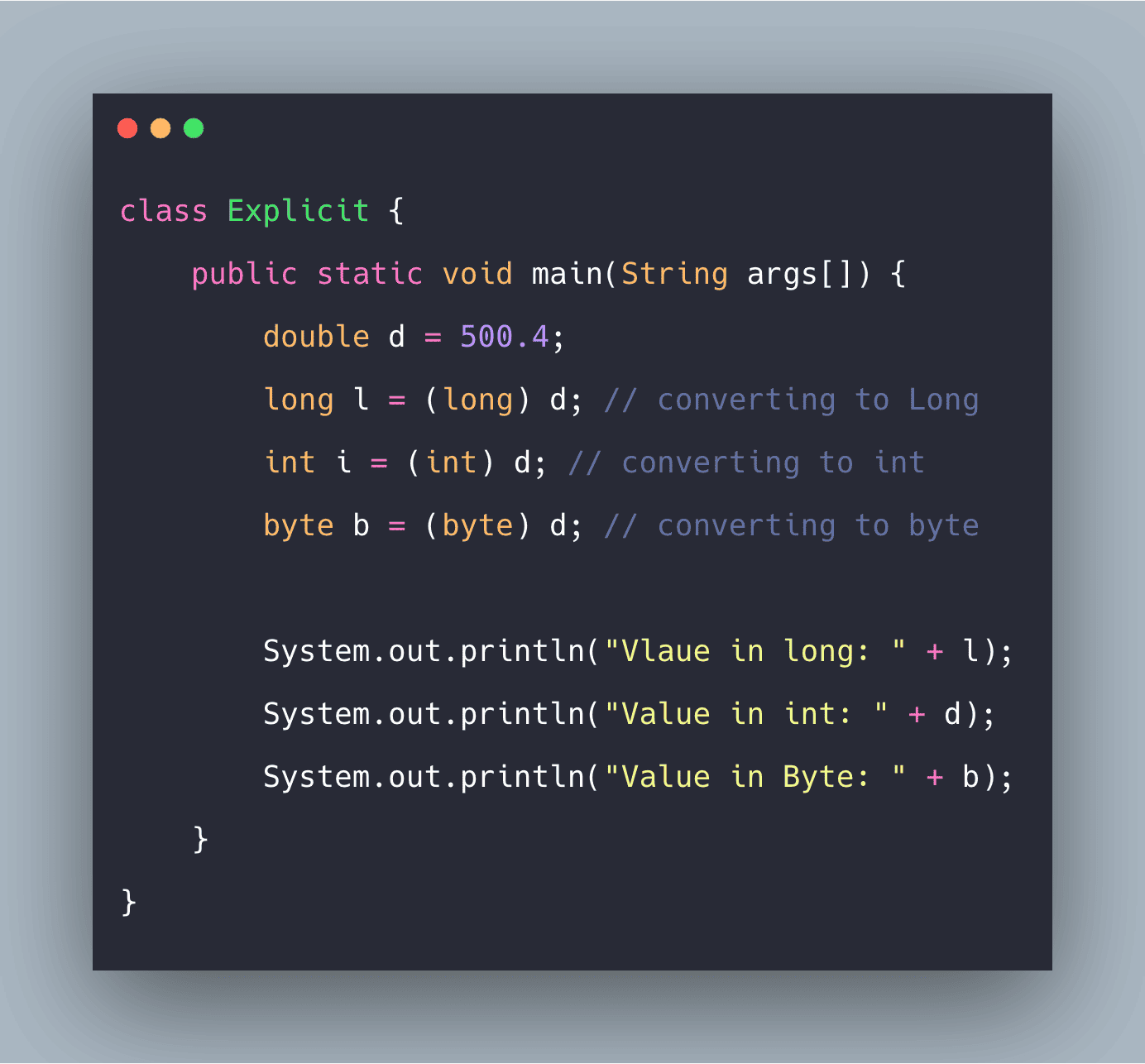Let’s say you want to assign the value of one data type into another variable of another data type, so there might be a case that they are not compatible with each other. To overcome this problem, we have one solution that we are going to learn today, called ‘Type Casting’ In Java, if both data types are compatible with each other, then it automatically has done its conversion, which is known as ‘Automatic type conversion.’

## Java Type Casting

Typecasting is used to convert an object or variable of one type into another.

There are two types of typecasting in Java they are:

1. Implicit Type Casting, known as Widening Type Casting
2. Explicit Type Casting, also known as Narrowing Type Casting

We will learn about these two types of typecasting.

## #Implicit Typecasting

This typecasting comes when the system automatically converts the data type of one variable into another if they are compatible. In other words, in this typecasting, the system only can convert the lower range to a higher range.

For example, if we have one variable int x=10, and we want to convert it into a long data type, we have to assign the value like this, long num=x simply. The system will automatically convert the value from int to long.

Always remember, in java we only can convert numeric type data type to another numeric type data type, means int to long or byte to int, etc. See the below chart from lower range to upper range of data type.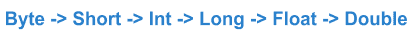See the below programming example of how the Widening or Automatic or Implicit type casting is done.

```class Implicit {
public static void main(String args[]) {
int b = 150;
// now we will convert it into long, and double
long l = b;
double d = b;
System.out.println("Long value is " + l);
System.out.println("Double value is " + d);
}
}
```

See the following output.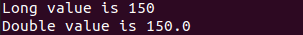## #Explicit Type Casting

Now suppose we have one variable which has a larger data type, let suppose in long, and we want it to be assigned to int. To do so, we have to apply explicit typecasting, also known as Narrowing Type Casting.

Note that this is not automatic typecasting, we have to do explicit type casting manually.  To do so, we have to write code by following the below syntax.

```new_operand = (new_data_type) current_operand;
```

For example, suppose we want to convert long x=555; to int num, so we have to write code like the following.

```long x=555;
int num;
num = (int) x;```

See the below diagram to know how we can apply explicit typecasting in the same type of data.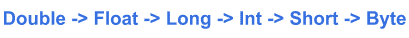So, from the above diagram, we can see that the flow of explicit type casting is precisely the opposite of Implicit Type Casting.  Now we will see one example of explicit typecasting and how it works.

```class Explicit {
public static void main(String args[]) {
double d = 500.4;
long l = (long) d; // converting to Long
int i = (int) d; // converting to int
byte b = (byte) d; // converting to byte

System.out.println("Vlaue in long: " + l);
System.out.println("Value in int: " + d);
System.out.println("Value in Byte: " + b);
}
}```

See the following output.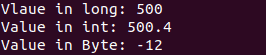From the above code, we can see that when we are going to convert the value of double to byte, it showing different values. Because the double data type has a maximum range of up to 0 to 255, so it reduced the number to modulo 256.

Also, note that you cannot convert a char value to int, because they are not compatible with each other.

While evaluating the expressions, an intermediate value may exceed the range of operands, and hence, an expression value will be promoted. Some conditions for type promotion are:

1. Java automatically promotes each byte, short, or char operand to int when evaluating the expression.
2. If one operand is a long, float or double, the whole expression is promoted to long, float or double respectively.

So here, Java Type Casting Example Tutorial is over.

This site uses Akismet to reduce spam. Learn how your comment data is processed.